# 8 Results

View
Selected filters:
• Ratios and Proportions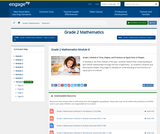Conditions of Use:
Remix and Share
Rating

In Module 8, the final module of the year, students extend their understanding of partåÐwhole relationships through the lens of geometry.åÊ As students compose and decompose shapes, they begin to develop an understanding of unit fractions as equal parts of a whole.

Subject:
Mathematics
Ratios and Proportions
Material Type:
Module
Provider:
New York State Education Department
Provider Set:
EngageNY
02/01/2014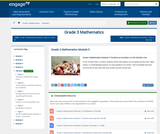Conditions of Use:
Remix and Share
Rating

In this 35-day Grade 3 module, students extend and deepen second grade practice with "equal shares" to understanding fractions as equal partitions of a whole. Their knowledge becomes more formal as they work with area models and the number line.

Subject:
Mathematics
Ratios and Proportions
Material Type:
Module
Provider:
New York State Education Department
Provider Set:
EngageNY
02/03/2013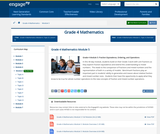Conditions of Use:
Remix and Share
Rating

In this 40-day module, students build on their Grade 3 work with unit fractions as they explore fraction equivalence and extend this understanding to mixed numbers.åÊ This leads to the comparison of fractions and mixed numbers and the representation of both in a variety of models.åÊ Benchmark fractions play an important part in studentsåÕ ability to generalize and reason about relative fraction and mixed number sizes.åÊ Students then have the opportunity to apply what they know to be true for whole number operations to the new concepts of fraction and mixed number operations.

Subject:
Mathematics
Ratios and Proportions
Material Type:
Module
Provider:
New York State Education Department
Provider Set:
EngageNY
01/17/2014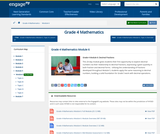Conditions of Use:
Remix and Share
Rating

This 20-day module gives students their first opportunity to explore decimal numbers via their relationship to decimal fractions, expressing a given quantity in both fraction and decimal forms.åÊ Utilizing the understanding of fractions developed throughout Module 5, students apply the same reasoning to decimal numbers, building a solid foundation for Grade 5 work with decimal operations.

Subject:
Mathematics
Ratios and Proportions
Material Type:
Module
Provider:
New York State Education Department
Provider Set:
EngageNY
02/01/2014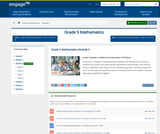Conditions of Use:
Remix and Share
Rating

In Module 3, students' understanding of addition and subtraction of fractions extends from earlier work with fraction equivalence and decimals. This module marks a significant shift away from the elementary grades' centrality of base ten units to the study and use of the full set of fractional units from Grade 5 forward, especially as applied to algebra.

Subject:
Mathematics
Ratios and Proportions
Material Type:
Module
Provider:
New York State Education Department
Provider Set:
EngageNY
11/27/2012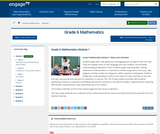Conditions of Use:
Remix and Share
Rating

Students begin their sixth grade year investigating the concepts of ratio and rate. They use multiple forms of ratio language and ratio notation, and formalize understanding of equivalent ratios. Students apply reasoning when solving collections of ratio problems in real world contexts using various tools (e.g., tape diagrams, double number line diagrams, tables, equations and graphs). Students bridge their understanding of ratios to the value of a ratio, and then to rate and unit rate, discovering that a percent of a quantity is a rate per 100. The 35 day module concludes with students expressing a fraction as a percent and finding a percent of a quantity in real world concepts, supporting their reasoning with familiar representations they used previously in the module.

Subject:
Mathematics
Ratios and Proportions
Material Type:
Module
Provider:
New York State Education Department
Provider Set:
EngageNY
05/14/2013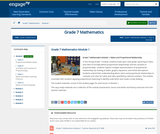Conditions of Use:
Remix and Share
Rating

In this 30-day Grade 7 module, students build upon sixth grade reasoning of ratios and rates to formally define proportional relationships and the constant of proportionality.åÊ Students explore multiple representations of proportional relationships by looking at tables, graphs, equations, and verbal descriptions.åÊ Students extend their understanding about ratios and proportional relationships to compute unit rates for ratios and rates specified by rational numbers. The module concludes with students applying proportional reasoning to identify scale factor and create a scale drawing.

Subject:
Mathematics
Ratios and Proportions
Material Type:
Module
Provider:
New York State Education Department
Provider Set:
EngageNY
05/14/2013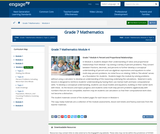Conditions of Use:
Remix and Share
Rating

In Module 4, students deepen their understanding of ratios and proportional relationships from Module 1 by solving a variety of percent problems. They convert between fractions, decimals, and percents to further develop a conceptual understanding of percent and use algebraic expressions and equations to solve multi-step percent problems. An initial focus on relating 100% to åÒthe wholeåÓ serves as a foundation for students.åÊ Students begin the module by solving problems without using a calculator to develop an understanding of the reasoning underlying the calculations.åÊ Material in early lessons is designed to reinforce studentsåÕ understanding by having them use mental math and basic computational skills. To develop a conceptual understanding, students use visual models and equations, building on their earlier work with these.åÊ As the lessons and topics progress and students solve multi-step percent problems algebraically with numbers that are not as compatible, teachers may let students use calculators so that their computational work does not become a distraction.

Subject:
Mathematics
Ratios and Proportions
Material Type:
Module
Provider:
New York State Education Department
Provider Set:
EngageNY